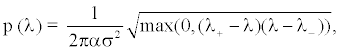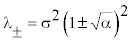﻿ Information or Noise? That is the Question
Journal of Health & Medical Informatics

Like us on:

• Editorial
• J Health Med Informat 2013, Vol 4(3): e107
• DOI: 10.4172/2157-7420.1000e107

# Information or Noise? That is the Question

Yu Ding*
Dorothy M. Davis Heart and Lung Research Institute, The Ohio State University, Columbus, OH, 43210, USA
*Corresponding Author: Yu Ding, Dorothy M. Davis Heart and Lung Research Institute, The Ohio State University, 326 BRT, 460 West 12th Avenue, Columbus, OH43210, USA, Tel: (614)247-7376, Fax: (614)247-8277, Email: [email protected]

Received Date: Jul 15, 2013 / Accepted Date: Jul 16, 2013 / Published Date: Jul 20, 2013

### Abstract

#### Introduction

What is signal and what is noise? That is the question of health and medical data processing. An interesting subjective answer is: “one man´s noise is another man´s signal”. But most researchers in this area are looking for an objective answer that can separate signal from noise based on an objective metric. Most people believe that the metric should be a statistical measure because the noise has high randomness, and the signal has spatial-temporal structure. Alternatively, if a proper linear transform is applied to the noise-corrupted data, then signal can be mapped into a sub-space, and noise is still uniformly distributed in the whole vector space. Therefore, signal is compressible, but noise is not. If the transform is a unitary transform, e.g. Fourier transform, then the noise variance remains the same. Based on this assumption, most of the de-noising processes have three generic steps: 1. Transform the data to a transform domain, e.g. spatial/temporal Fourier domain, wavelet domain etc.; 2. Truncation in the transform domain by removing/ suppressing noise-only or noise-dominate modes; 3. Inverse transform to obtain the de noised data. However, there is still no generic method to find the optimal truncation threshold. Application specific/empirical criteria are usually applied. One of the widely-used linear transforms in signal processing and artificial intelligence is the principal component analysis (PCA), a.k.a. Karhunen-Loeve transform, Hotelling transform and proper orthogonal decomposition. It is an adaptive unitary transform, which is optimal in the least square sense . Numerous empirical eigenmode selection metrics were proposed, e.g. knee point, parallel analysis . Again, there is no well-established generic threshold selection rule based on solid statistical theory .

Recently, a new threshold selection method was proposed, and it has the potential to be a generic method independent of any specific application/data type [2,3]. This new method has only one assumption: the data matrix is a sum of a low-rank signal matrix and a full-rank noise matrix. Then the new eigenmode selection method was derived based on the random matrix theory.

The so-call random matrix is a matrix with random entries, e.g. a noise matrix. Physicist proposed this concept in the 1950´s, and utilized it to explain the gap in nuclear energy levels . Theoretical physicists worked with RMT since then , but did not study the most important feature of the RMT-the empirical eigenvalue probability distribution function (PDF). Two mathematicians from the Russian school, Marcenko and Pastur , first published the empirical eigenvalue PDF (so-called MP-law) in 1967. It was unknown to the western math society, until the late 1990´s [7,8]. The MP-law states that: if the data matrix is a random matrix with each entry an independent and identically distributed (IID) random variable, then the eigen values (λ) of the corresponding Wishart matrix asymptotically follow the following probability distribution function:(1)

Where α=r/n,, and n are two dimensions of the random matrix, σ is the noise standard deviation. Please note that the MP-law distribution function is continuous but not differentiable. λ+ λ- and are theupper and the lower bound.

In 1999, two physicists, Sengupta and Mitra , published their results when both signal and noise appear in the data matrix, i.e., if the data matrix is a sum of a low-rank signal matrix and a full-rank noise matrix, then the eigen values corresponding to the null-space of the signal matrix still follow the MP-law . This important resultimplies the following two points: 1) the PCA can map the data into two subspaces: the signal dominated sub-space and the noise-dominated subspace; 2) the eigenvalues of the noise-dominated sub-space still follow the MP-law. Therefore, the noise-dominated sub-space can be found by identifying which eigenvalues follow the MP-law. This is a generic approach to select the eigenmode threshold only based on statistical property. Several theoretical studies proposed this approach, but none of them demonstrated the effectiveness of this approach using data collected in the real-world [10-12].

The reason is simple but subtle: the MP-law is very sensitive to the correlation in the noise. All electrical circuits cannot have ideal impulse response function (Dirac delta function). Therefore, even random fluctuations show some degree of correlation, so does the noise in all raw data collected by sensors. Hence, there is no such a thing called IID noise in the real-world. Another layer of complexity comes from the construction of the data matrix: the noise may have correlation along both the row dimension and the column dimension. Therefore, the noise correlation in the data matrix is a 4th order tensor. There is no closed-form expression of empirical eigenvalue PDF of a random matrix with a generic noise correlation tensor.

Even the complex noise correlation is problematic; the MP-law based method can be salvaged. When the noise is non-IID, eigen values corresponding to the noise-only eigenmodes follows the MP-law with modified parameter(s). Intuitively, because of the noise correlation, the degree of freedom in the noise data should be reduced to a lower value, i.e. parameter n in Equation  should be modified to n´<n. Then, the noise-dominated eigenmodes can be identified by maximizing the goodness-of-fit between the eigenvalues and the MP-law with modified parameter n [2,3]. This is so-called the MP-law method. The new method has been proven to be effective in the dynamic MRI data using parallel imaging reconstruction, which is corrupted by complex non- IID noise [13,14]. Potentially, the MP-law method can be utilized as a generic PCA eigenmode selection metric.

The recently developed MP-law method should be regard as an engineer´s solution of the PCA eigenmode selection problem. It is a practical approach to answer the ubiquitous question: “what is information and what is noise”, whenever PCA is utilized. Its effectiveness has been tested using medical imaging data. But there is a missing link between the correlation tensor and the accuracy of the MP-law method. We hope mathematicians will bridge up the missing link in the near future, and hence, provide a more powerful random matrix toolbox for the health and medical data processing.

#### References

Citation: Ding Y (2013) Information or Noise? That is the Question. J Health Med Informat 4:e107. Doi: 10.4172/2157-7420.1000e107

Copyright: © 2013 Ding Y. This is an open-access article distributed under the terms of the Creative Commons Attribution License, which permits unrestricted use, distribution, and reproduction in any medium, provided the original author and source are credited.

Select your language of interest to view the total content in your interested language

##### Recommended Journals
Viewmore
###### Article Usage
• Total views: 12223
• [From(publication date): 10-2013 - Mar 22, 2019]
• Breakdown by view type
• HTML page views: 8428

## Post your commentCan't read the image? click here to refresh
###### Peer Reviewed Journals

Make the best use of Scientific Research and information from our 700 + peer reviewed, Open Access Journals

International Conferences 2019-20

Meet Inspiring Speakers and Experts at our 3000+ Global Annual Meetings

Top